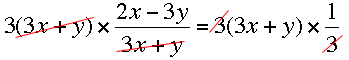SEARCH HOMEMath Central Quandaries & QueriesQuestion from Yvonne, a parent: Hi, Would you please help me on the following 2 questions? 1. Given that 3x-5y / 2x-y = 2/3, find the value of 2x/5y. 2. If 3x-5y / 7x-4y = 3/4, find the value of x/y. Thank you.Yvonne,

(2x - 3y)/(3x + y) = 1/3 find the value of 2x/5y.

I would start by multiplying both sides of (2x - 3y)/(3x + y) = 1/3 by 3(3x + y) to eliminate the fractionsor

3(2x - 3y) = 3x + y

which simplifies to

3x = 10 y

thus

x/y = 10/3 so 2x/5y = 2/5 × 10/3 = 4/3